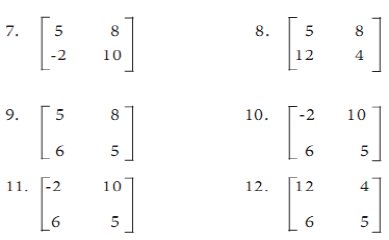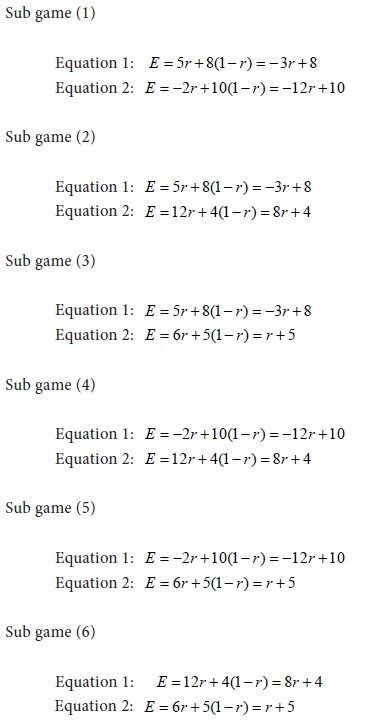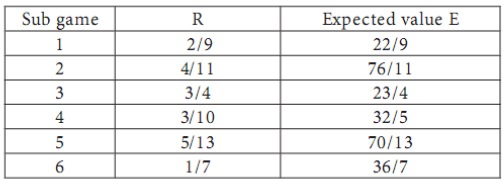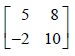Home | ARTS | Operations Management | The concept and Approaches for m x 2 zero-sum game

# The concept and Approaches for m x 2 zero-sum game

Posted On :  25.06.2018 01:06 am

When the second player B has exactly two strategies and the first player A has m (where m is three or more) strategies, there results an m x 2 game.

The concept of an m x 2 zero-sum game

When the second player B has exactly two strategies and the first player A has m (where m is three or more) strategies, there results an m x 2 game. It is also called a rectangular game. Since B has two strategies only, he will find it difficult to discard any one of them. However, since A has more strategies, he will be in a position to make out some choice among them. He can retain some of the most advantageous strategies and give up some other strategies. The motive of A is to get as maximum payoff as possible. Therefore, if some strategies are available to A by which he can get more payoff to himself, then he will retain such strategies and discard some other strategies which result in relatively less payoff.

## Approaches for m x 2 zero-sum game

There are two approaches for such games: (1) Sub game approach and (2) Graphical approach.

### Sub game approach

The given m x 2 game is divided into 2 x 2 sub games. For this purpose, consider all possible 2 x 2 sub matrices of the payoff matrix of the given game. Solve each sub game and have a list of the values of each sub game. Since A can make out a choice of his strategies, he will be interested in such of those sub games which result in more payoff to himself. On the basis of this consideration, in the long run, he will retain two strategies only and give up the other strategies.

Problem

Solve the following gameSolution

Let us consider all possible 2x2 sub games of the given game. We have the following sub games:Let E be the expected value of the payoff to player A. i.e., the loss to player B.    Let r be the probability that player B will use his first strategy. Then the probability that he will use his second strategy is 1-r. We form the equations for E in all the sub games as follows:Solve the equations for each 2x2 sub game. Let us tabulate the results for the various sub games. We have the following: Sub gameInterpretation

Since player B has only 2 strategies, he cannot make any choice on his strategies. On the other hand, player A has 4 strategies and so he can retain any 2 strategies and give up the other 2 strategies. Since the choice is with A, he will try to maximize the payoff to himself. The pay-off to A is the maximum in the case of sub game 1. i.e., the sub game with the matrix .Therefore, player A will retain his strategies 1 and 2 and discard his strategies 3 and 4, in the long run. In that case, the probability that B will use his first strategy is r = 2/9 and the probability that he will use his second strategy is 1-r = 7/9 . i.e., Out of a total of 9 trials, he will use his first strategy two times and the second strategy seven times.

The value of the game is 22/3 . The positive sign of V shows that the game is favourable to player A.

Tags : Operations Management - Game Theory, Goal Programming & Queuing Theory
Last 30 days 965 views